# An Efficient ASIC Design for SHA256

## Introduction

A hash function is used to compress arbitrary message into a fixed length hash result. For one hash function, when given the same information, it always provides the same hash value. Thus, hash functions can be used to verify that the message remains identical during transmission or other processes, without comparing the message bitwise. Secure hash algorithms (SHA) introduced by the National Institute of Standards and Technology (NIST) are hash functions that are widely used in all kinds of applications.

Recently, encrypted currencies become quite popular, and the price of the famous bitcoin grew dramatically. One problem in the Bitcoin economy is about the mining process, which generates new Bitcoins. This process is mostly about finding the original string corresponding to a given SHA-256 result. But since hash calculations are irreversible, there’s no solution other than computing the SHA-256 value of every possible string. That’s why efficiently executing SHA-256 is one of the significant challenges in Bitcoin mining. Results suggest developing efficient ASIC unit dedicated to the SHA-256 algorithm is the best solution. Companies in the field, like Bitmain and Canaan, provides machines that can calculate several trillion hash blocks per second, while the latest Nvidia GPU can only calculate several billion hash blocks per second.

In this article, I will include the basic design of an ASIC unit for SHA-256 base on the article “The Design of a High Speed ASIC Unit for the Hash Function SHA-256 (384, 512)” by Dadda and Macchetti. The design will be base on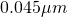and compared with the result provided in the article.

## Design Detials

### The algorithm and the schemes

According to the standard by NIST, SHA-256 is shown as Algorithm 1. Because the pre-processing procedure (Line 2) is only calculated once for every message, it’s costly to implement hardware of this part. Thus, my design will input pre-processed data rather than raw data.

 Algorithm 1: SHA-256 Require: Variables are 32 bit unsigned integers in default.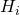: Hash value,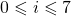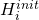:initial value,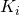: Constant,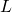: Length of the input (in bit), 64 bit unsigned integer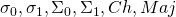: Predefined logic functions: Append operation ENSURE: Go through the whole iteration for every message 1.  Initialize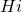with2.  Pre-processing: Append one ‘1’ bit,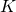‘0’ bit and, such that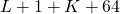is a multiple of 512 3.  Divide the message into 512 bit chunk 4.  for every chunk do 5.      Divide into sixteen 32 bit integers (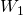to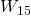) 6.      for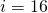to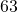do 7.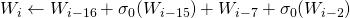8.      end for 9.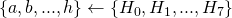10.    for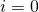todo 11.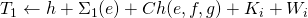12.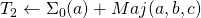13.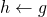14.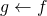15.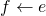16.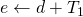17.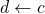18.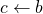19.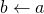20.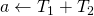21.     end for 22.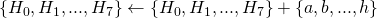23. end for 24. print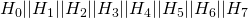The architecture of the ASIC unit is shown as Figure 1. Hash Value Generator is the unit related to Line 4 to Line 23 in Algorithm 1. This module generates hash values from the message and will be implemented using three stage pipeline. Output Control is designed to output hash value (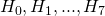) by 32 bit per clock cycle. CALCU_EN is the input signal that indicates the start of a new hash block. CALCU_RDY is the output signal that indicates the finish of a hash block. READ_EN is the input signal that indicates the start of hash value output.Figure 1: Architecture of SHA-256 ASIC Unit.

It’s obvious that the Hash Value Generator is the key point of the design. The scheme of this module is shown in Figure 2. In the design, Full Adder Arrays (FAA) are used to speed up the add operations. Each FAA unit is a 3:2 compressor, in which the carryout of a one-bit adder is taken as the output rather than add to the next bit. This guarantees that all bits are independent and can be calculated in parallel. Becauseare logic operations, they are much faster than both FAA and adder, their delays can be safely ignored. And since an adder with Carry Look Ahead (CLA) is at least three times slower than an FAA, the critical path of pipeline in the shown scheme are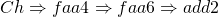and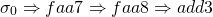The clock frequency will be chosen base on these critical paths.Figure 2: Scheme of the Hash Value Generator.

### Time Diagram

There are basically two operations, including the calculation part, in which the ASIC unit calculates the SHA256 value for a given block, and the output part, in which the ASIC unit outputs the calculation result.Figure 3: The timing diagram of the calculation part for SHA256 ASIC unit

The timing diagram of the calculation part is shown in Figure 3. If this is the start of a new SHA256 calculation, the RST signal will be set high to clear all the memory. Then, CALCU_EN signal will be set high to start the calculation of a block. The word to calculate will be input sequentially, which will last 16 clock cycles. At last, after 67 clock cycles, the CALCU_RDY signal will be set high by the ASIC to indication the end of the calculation, and the calculation of another block shall begin at the next clock cycle.Figure 4: The timing diagram of the output part for SHA256 ASIC unit

The timing diagram of the output part is shown in Figure 4. After finishing all the calculation, the READ_EN signal will be set high to indicate the start of a read operation. Then, the 64-bit hash value will be output sequentially in the next 8 clock cycles.

## Implement

To be continued…

Reference

 Bitmain. Antminer s9-14th/s with psu. [Online]. Available: https://shop.bitmain.com/product/detail?pid=00020180327210606975nMDzruwu0747

 J. M. Gosney. 8x nvidia gtx 1080 hashcat benchmarks. [Online]. Available: https://gist.github.com/epixoip/a83d38f412b4737e99bbef804a270c40

 L. Dadda, M. Macchetti, and J. Owen, “The design of a high speed ASIC unit for the hash function SHA-256 (384, 512),” in Proceedings Design, Automation and Test in Europe Conference and Exhibition. IEEE Comput. Soc, 2004.

 NIST, “180-2 federal information processing standards publication,” SECURE HASH STANDARD, National Institute of Standards and Technology, 2002.

 L. Bai and S. Li, “VLSI implementation of high-speed SHA-256,” in 2009 IEEE 8th International Conference on ASIC. IEEE, oct 2009